## Energetic Costs of Partial State Excitation of Molecular Oxygen by Auroral Electrons

The costs for initial electron energies 100 eV, 1 keV and 10 keV were calculated for air at ~120 km (mixture of 70% N2, 10% O2 and 20% O).

#

State

Threshold, eV

Eo=100eV

Eo=1keV

Eo=10keV

Plot for Eo<50eV

With e-e collisions

1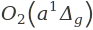0.98

12.93

14.44

14.59

View plot

View plot

21.63

36.15

40.32

40.66

View plot

View plot

3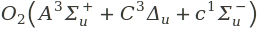4.5

98.98

109.9

110.8

View plot

View plot

47.1

50.19

60.14

61.46

View plot

View plot

5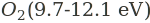9.7

420

530.8

550.3

View plot

View plot

6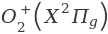12.1

400.1

399.8

418.3

View plot

View plot

716.1

243.8

199.8

203.9

View plot

View plot

8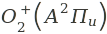16.9

253.9

206.4

209.8

View plot

View plot

9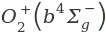18.2

346.8

258.2

259.6

View plot

View plot

10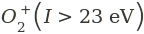23

1016

539.4

520.7

View plot

View plot

1118.9

179.6

141.6

147.7

View plot

View plot